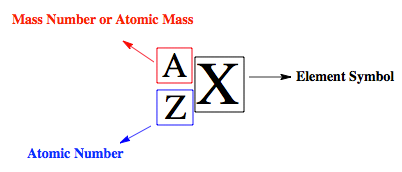# Problem: Which of the following sets represents a pair of isotopes? 14C and 14N 206Pb and 208Pb O2 and O3 32S and 32S2-

###### FREE Expert Solution

Isotopes represent atoms of an element that contain the same atomic number (same number of protons), but different mass numbers (different number of neutrons).

The notation used for isotopes is shown below:Recall that the mass number or atomic mass of an element represents the total number of its protons and neutrons, while the atomic number represents the total number of its protons.

88% (69 ratings)###### Problem Details

Which of the following sets represents a pair of isotopes?

14C and 14N

206Pb and 208Pb

O2 and O3

32S and 32S2-